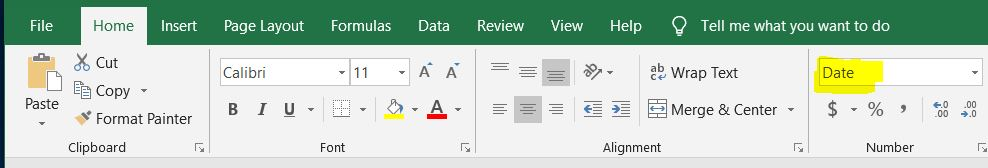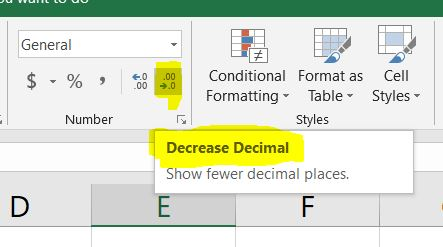## How do you calculate age using a formula or function in Excel

To calculate age in Excel is relatively easy if you know the proper function to use. In this quick example I am going to use the today() function to perform some simple date math.

In the below example we have a column of data with a birthday.The Birthday column is formatted as “Date”.  Excel should automatically change this from the default format of  “General” but you can confirm by clicking on the cell and checking the Home tab, then the Number group, and then check the dropdown box.In logical human terms your age is today date minus the date you were born. This will calculate the number of days you have been alive. We then need to take that number and dived by the number of days in a year (365).

The formula in Excel looks like this.

`=(TODAY()-A2)/365`

A2 is the reference to the cell containing the birthday. The function “today()” is a built in Excel function that always references the current date. See the screen shot below.## Formatting the number of decimal places

The result is returned with more decimal places than we probably need. If you would like to decrease the decimal places you can do so by selecting the entire row containing the age then clicking the icon on the Home tab, Number group shown below.If you would only like to calculate age to the nearest year you can reduce the decimal places to none. The result is shown below.## Summary

Calculating an age is not difficult if done properly in Excel. Using the today() function in Excel will keep the worksheet updated every time the document is opened. If you open the same document a year from now each rows age will be increased by one. This may be preferred over manually updating ages by hand.  This type of date math can also be used to calculate years of service with a company. The formula could use a Hired Date column rather than a Birthday.